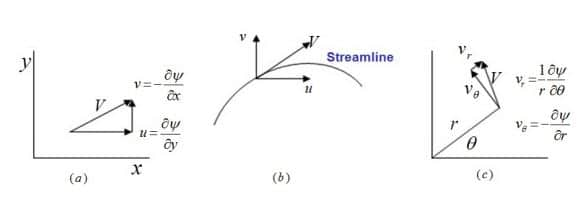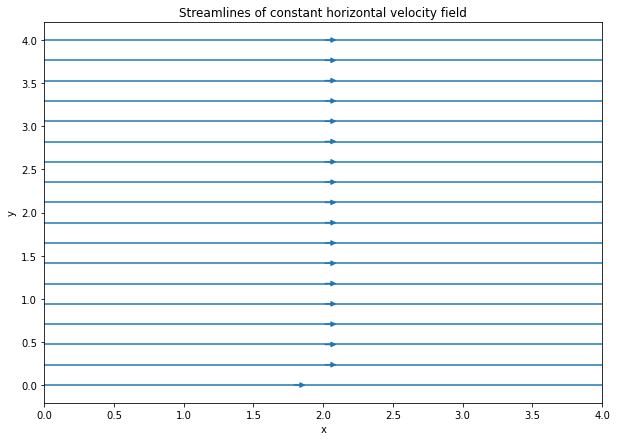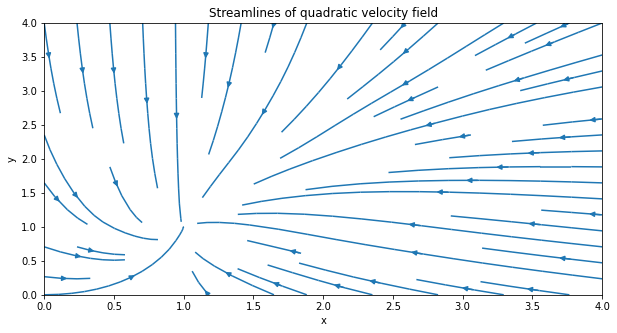# Stream functions and stream lines#

Geophysical Fluid Dynamics of the Oceans

In this notebook, stream functions and streamlines will be explained and visualised.

import numpy as np
import matplotlib.pyplot as plt
import plotly.figure_factory as ff
import plotly.graph_objects as go


A stream function, $$\psi$$, is a scalar function of which the derivative gives the velocity field at right angles to the stream function. The stream function is a function of space $$(x,y)$$ and time and is defined as $$\psi = f(x,y)$$.

$\frac{\partial \psi}{\partial x} = v$
$\frac{\partial \psi}{\partial y} = -u$

where $$u(x,y)$$ and $$v(x,y)$$ are velocity component functions.The stream function satisfies the continuity equation and is therefore incompressible. This means that

$\nabla (u,v) = \frac{\partial u}{\partial x} + \frac{\partial v}{\partial y} = \frac{\partial^2 \psi}{\partial x \partial y} + \frac{\partial^2 \psi}{\partial y \partial x}$

It can be both rotational and irrotational. It is irrotational if it satisfies Laplace’s equation of the form

$\frac{\partial^2 \psi}{\partial x^2} + \frac{\partial^2 \psi}{\partial y^2} = 0$

Streamlines are the lines along which the stream function is constant, so along a streamline $$d \psi = 0$$. A few properties of streamlines are:

• All the streamlines are parallel to each other

• With a constant stream function value, infinite amounts of streamlines can be drawn

In Python, streamlines can be visualised using the Streamplot within matplotlib that draws the streamlines of a vector field.

# creating a spatial grid
x = np.arange(0, 5)
y = np.arange(0, 5)
X, Y = np.meshgrid(x, y)

# creating components of the velocity field
u = np.ones((5, 5)) # x-component, zeroes
v = np.zeros((5, 5)) # y-component

fig = plt.figure(figsize = (10, 7))

plt.title('Streamlines of constant horizontal velocity field')
plt.xlabel('x')
plt.ylabel('y')
# Plotting stream plot
# density dictates how closely spaced the streamlines are plotted
plt.streamplot(X, Y, u, v, density = 0.6)

<matplotlib.streamplot.StreamplotSet at 0x23ff27a7340>Now, a stream function plot will be made for velocity components that vary within the grid. Now, the x-component of the velocity field, $$u$$, will be of the function $$u = 1-x^2$$ and the y-component of the field, $$v$$, will be defined as $$v = x - y^2$$.

x = np.arange(0, 5)
y = np.arange(0, 5)
X, Y = np.meshgrid(x, y)

U = 1 - X**2
V = X - Y**2

fig = plt.figure(figsize = (10, 5))
plt.xlabel('x')
plt.ylabel('y')
plt.streamplot(X, Y, U, V, density = 0.6)

<matplotlib.streamplot.StreamplotSet at 0x23ff2961a60>A more interactive (move cursor over plot to see coordinates) and other good way to create a stream function plot, is by using figure factory from the Plotly module.

Moreover, a source point can be created using Graph_objects from the Plotly module. Below is shown how this can be plotted. Here, the velocity field $$u$$ and y include the source components in their function.

# create spatial meshgrid
x = np.linspace(0, 5, 100)
y = np.linspace(0, 5, 100)
X, Y = np.meshgrid(x, y)

# define source strength and location
source_strength = 10
x_source, y_source = 2, 2

# define velocity field as function X and Y
u = (source_strength *
(X - x_source)/((X - x_source)**2 + (Y - y_source)**2))
v = (source_strength*
(Y - y_source)/((X - x_source)**2 + (Y - y_source)**2))

# Create streamline figure
fig = ff.create_streamline(x, y, u, v,
name='Stream line')